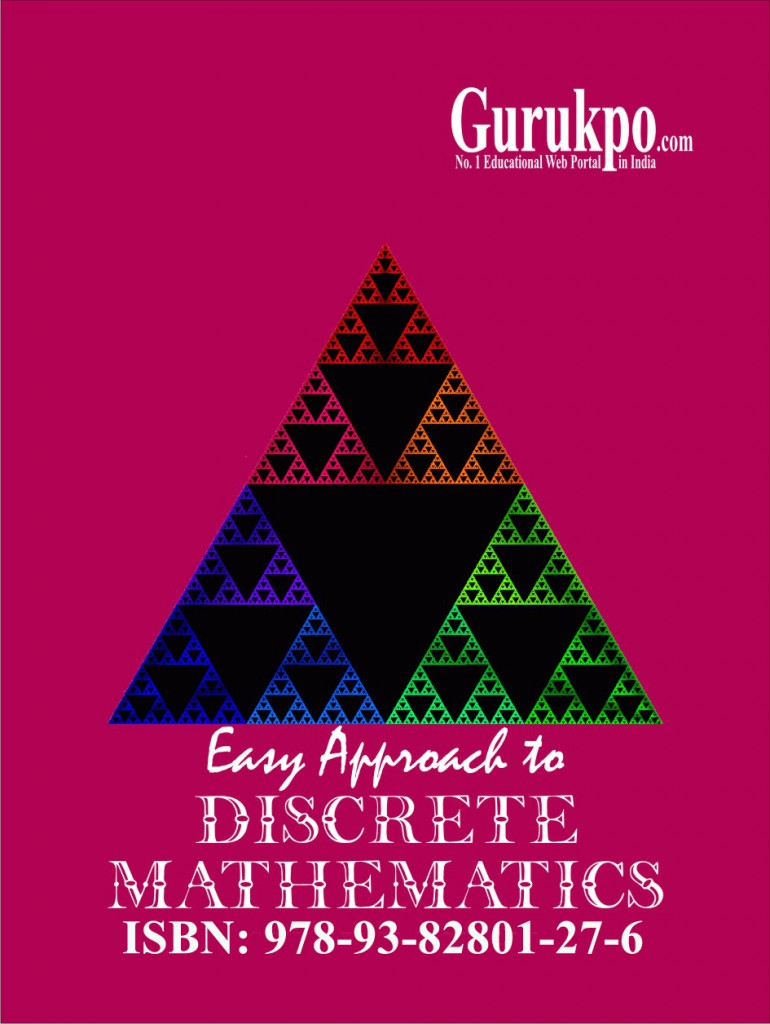## Discrete Mathematics

Introduction to Discrete Mathematical Structures, Formal Methods: Introduction and Analogy, Abstraction. Fundamentals: Sets & Relations- Sets, Types of Sets, Multi Sets, Operations on Sets, Relations and Properties of Relations, Representation of Relations, Equivalence Relation, Closures of Relations, Methods of Proof-Direct Proofs, Indirect Proofs, Mathematical Induction, Method of Contradiction. Combinatorics: Permutations and Combinations, Pigeon Hole Principle, …